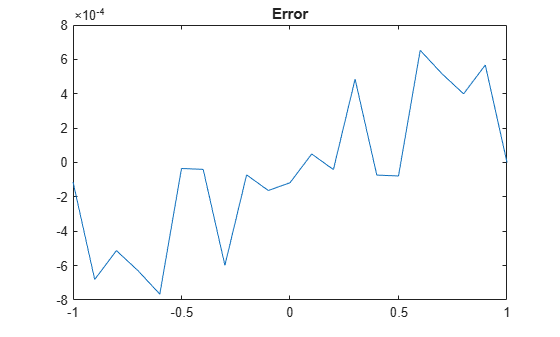# cordicacos

CORDIC-based approximation of inverse cosine

Since R2018b

## Syntax

``theta = cordicacos(x)``
``theta = cordicacos(x,niters)``

## Description

example

````theta = cordicacos(x)` returns the inverse cosine of `x` based on a double CORDIC (DCORDIC) algorithm approximation.```

example

````theta = cordicacos(x,niters)` performs `niters` iterations of the DCORDIC algorithm.```

## Examples

collapse all

Compute the inverse cosine of a fixed-point `fi` object using a CORDIC implementation.

```a = fi(-1:.1:1,1,16); b = cordicacos(a); plot(a,b); title('Inverse CORDIC Cosine');```Compare the output of the `cordicacos` function and the `acos` function.

```c = acos(double(a)); error = double(b)-c; plot(a,error); title('Error');```Find the inverse cosine of a `fi` object using a CORDIC implementation and specify the number of iterations the CORDIC kernel should perform. Plot the CORDIC approximation of the inverse cosine with varying numbers of iterations.

```a = fi(-1:.1:1,1,16); for i = 5:5:20 b = cordicacos(a,i); plot(a,b); hold on; end legend('5 iterations','10 iterations',... '15 iterations','20 iterations')```## Input Arguments

collapse all

Cosine of angle, specified as a scalar, vector, matrix, or multidimensional array. `x` must be a real, finite value in the range `[-1,1]`.

When `x` is specified as a `fi` object, the property `'DataTypeMode'` must equal `Fixed-point: binary point scaling'`, ```'Scaled double: binary point scaling'```, `'double'`, or `'single'`.

Data Types: `single` | `double` | `fi`
Complex Number Support: Yes

Number of iterations the CORDIC algorithm performs, specified as a positive integer-valued scalar.

If you do not specify `niters`, the algorithm uses a default value. For fixed-point inputs, the default value of `niters` is one less than the word length of the input array `x`. For double-precision inputs, the default value of `niters` is 54. For single-precision inputs, the default value is 25.

Data Types: `single` | `double` | `int8` | `int16` | `int32` | `int64` | `uint8` | `uint16` | `uint32` | `uint64` | `fi`

## Output Arguments

collapse all

Inverse cosine angle values in radians, returned as a scalar, vector, matrix, or multidimensional array. The `cordicacos` function returns values in the interval `[0,π]`.

collapse all

### CORDIC

CORDIC is an acronym for COordinate Rotation DIgital Computer. The Givens rotation-based CORDIC algorithm is one of the most hardware-efficient algorithms available because it requires only iterative shift-add operations (see References). The CORDIC algorithm eliminates the need for explicit multipliers. Using CORDIC, you can calculate various functions such as sine, cosine, arcsine, arccosine, arctangent, and vector magnitude. You can also use this algorithm for divide, square root, hyperbolic, and logarithmic functions.

Increasing the number of CORDIC iterations can produce more accurate results but also increases the expense of the computation and adds latency.

 Volder, Jack E. “The CORDIC Trigonometric Computing Technique.” IRE Transactions on Electronic Computers. EC-8, no. 3 (Sept. 1959): 330–334.

 Andraka, Ray. “A Survey of CORDIC Algorithm for FPGA Based Computers.” In Proceedings of the 1998 ACM/SIGDA Sixth International Symposium on Field Programmable Gate Arrays, 191-200. https://dl.acm.org/doi/10.1145/275107.275139.

 Walther, J.S. “A Unified Algorithm for Elementary Functions.” In Proceedings of the May 18-20, 1971 Spring Joint Computer Conference, 379-386. https://dl.acm.org/doi/10.1145/1478786.1478840.

 Schelin, Charles W. “Calculator Function Approximation.” The American Mathematical Monthly, no. 5 (May 1983): 317-325. https://doi.org/10.2307/2975781.